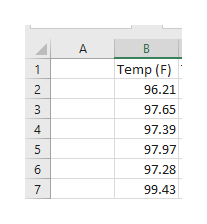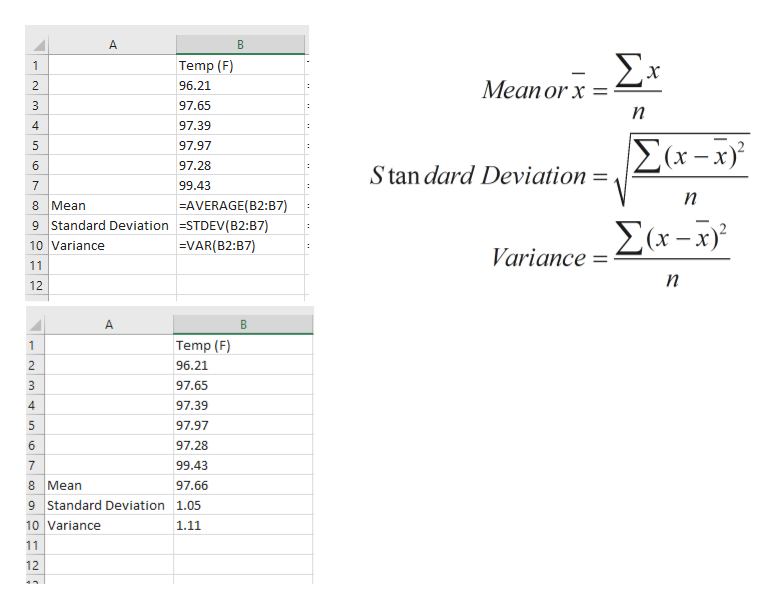# 6) Suppose you had the following body temperature readings, all in °F: (Don't forget to answer the questions in parts (d) and (e)).96.21 97.65 97.39 97.97 97.28 99.43a) calculate the mean, variance and standard deviation.b) now convert these readings to °C (us biologists like the metric system, after all). To convert, remember that °C = °F/1.8 - 32/1.8. For example, to convert the first reading you would do:96.21/1.8 - 32/1.8 = 53.43 - 17.78 = 35.67.c) now calculate the mean, variance and standard deviation of the converted data.d) take the mean you calculated in (a) and convert this to Celsius using the same formula you used in (b). Are you surprised by the results? Why or why not?e) take the standard deviation from (a) and divide it by 1.8. Can you explain the results? Why didn't you have to subtract 32/1.8? Watch your math in this step!

Question
68 views

6) Suppose you had the following body temperature readings, all in °F: (Don't forget to answer the questions in parts (d) and (e)).

96.21 97.65 97.39 97.97 97.28 99.43

a) calculate the mean, variance and standard deviation.

b) now convert these readings to °C (us biologists like the metric system, after all). To convert, remember that °C = °F/1.8 - 32/1.8. For example, to convert the first reading you would do:

96.21/1.8 - 32/1.8 = 53.43 - 17.78 = 35.67.

c) now calculate the mean, variance and standard deviation of the converted data.

d) take the mean you calculated in (a) and convert this to Celsius using the same formula you used in (b). Are you surprised by the results? Why or why not?

e) take the standard deviation from (a) and divide it by 1.8. Can you explain the results? Why didn't you have to subtract 32/1.8? Watch your math in this step!

check_circle

Step 1

As per guidelines, we are required to answer the first three subparts only. So I'll solve a,b and c

Begin by populating data into excel cells.help_outlineImage TranscriptioncloseB Temp (F) 1 2 96.21 97.65 4 97.39 5 97.97 97.28 7 99.43 fullscreen
Step 2

Calculate mean, standard deviation and variance using Excel functions as shown  ...help_outlineImage TranscriptioncloseA B Σx Temp (F) 1 Mean or x 2 96.21 97.65 3 n 97.39 Σα-3 97.97 97.28 6 Stan dard Deviation = 99.43 7 n AVERAGE(B2:B7) 9 Standard Deviation -STDEV ( B2: B7) 8 Mean Σα- (x 10 Variance =VAR(B2:B7) Variance 11 n 12 A Temp (F) 1 2 96.21 3 97.65 97.39 4 97.97 6 97.28 7 99.43 8 Mean 97.66 9 Standard Deviation 1.05 10 Variance 1.11 11 12 st LC fullscreen

### Want to see the full answer?

See Solution

#### Want to see this answer and more?

Solutions are written by subject experts who are available 24/7. Questions are typically answered within 1 hour.*

See Solution
*Response times may vary by subject and question.
Tagged in

### Data